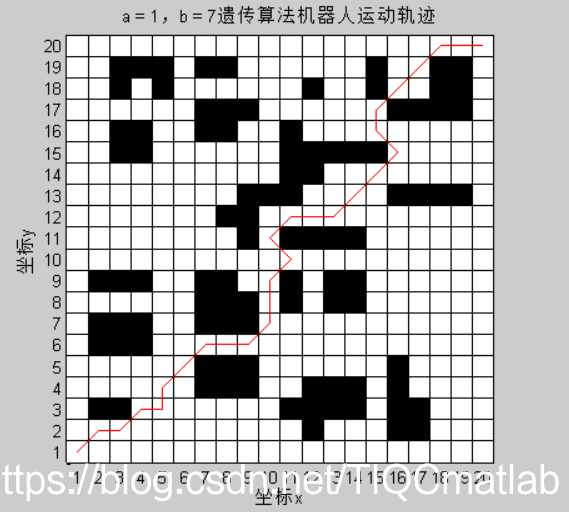# 【路徑規劃】基於matalb遺傳演算法機器人柵格地圖路徑規劃【含Matlab原始碼 022期】

1、種群初始化

2、選擇

3、交叉

4、變異

5、適應度計算

## 二、原始碼

```c % 基於遺傳演算法的柵格法機器人路徑規劃 clc; clear all close all; % 輸入資料,即柵格地圖 G= [0 0 0 0 0 0 0 0 0 0 0 0 0 0 0 0 0 0 0 0; 0 0 0 0 0 0 0 0 0 0 0 1 0 0 0 1 1 0 0 0; 0 1 1 0 0 0 0 0 0 0 1 1 1 1 0 1 1 0 0 0; 0 0 0 0 0 0 1 1 1 0 0 1 1 1 0 1 0 0 0 0; 0 0 0 0 0 0 1 1 1 0 0 0 0 0 0 1 0 0 0 0; 0 1 1 1 0 0 0 0 0 0 0 0 0 0 0 0 0 0 0 0; 0 1 1 1 0 0 1 1 1 0 0 0 0 0 0 0 0 0 0 0; 0 0 0 0 0 0 1 1 1 0 1 0 1 1 0 0 0 0 0 0; 0 1 1 1 0 0 1 1 0 0 1 0 1 1 0 0 0 0 0 0; 0 0 0 0 0 0 0 0 0 0 0 0 0 0 0 0 0 0 0 0; 0 0 0 0 0 0 0 0 1 0 1 1 1 1 0 0 0 0 0 0; 0 0 0 0 0 0 0 1 1 0 0 0 0 0 0 0 0 0 0 0; 0 0 0 0 0 0 0 0 1 1 1 0 0 0 0 1 1 1 1 0; 0 0 0 0 0 0 0 0 0 0 1 1 0 0 0 0 0 0 0 0; 0 0 1 1 0 0 0 0 0 0 1 1 1 1 1 0 0 0 0 0; 0 0 1 1 0 0 1 1 0 0 1 0 0 0 0 0 0 0 0 0; 0 0 0 0 0 0 1 1 1 0 0 0 0 0 0 1 1 1 1 0; 0 0 1 0 1 0 0 0 0 0 0 1 0 0 1 0 0 1 1 0; 0 0 1 1 1 0 1 1 0 0 0 0 0 0 1 0 0 1 1 0; 0 0 0 0 0 0 0 0 0 0 0 0 0 0 0 0 0 0 0 0]; ​ p_start = 0; % 起始序號 p_end =399; % 終止序號 NP = 200; % 種群數量 max_gen = 2000; % 最大進化代數 pc = 0.5; % 交叉概率 pm = 0.5; % 變異概率 a = 1; % 路徑長度比重 b = 7; % 路徑順滑度比重 %init_path = []; z = 1; new_pop1 = {}; % 元包型別路徑 [y, x] = size(G); % 起點所在列（從左到右編號1.2.3...） xs = mod(p_start, x) + 1; % 起點所在行（從上到下編號行1.2.3...） ys = fix(p_start / x) + 1; % 終點所在列、行 xe = mod(p_end, x) + 1; ye = fix(p_end / x) + 1; ​ % 種群初始化step1，必經節點,從起始點所在行開始往上，在每行中挑選一個自由柵格，構成必經節點 pass_num = ye - ys + 1; pop = zeros(NP, pass_num); min_value=1000; for i = 1 : NP pop(i, 1) = p_start; j = 1; % 除去起點和終點 for yk = ys+1 : ye-1 j = j + 1; % 每一行的可行點 can = []; for xk = 1 : x % 柵格序號 no = (xk - 1) + (yk - 1) * x; if G(yk, xk) == 0 % 把點加入can矩陣中 can = [can no]; end end can_num = length(can); % 產生隨機整數 index = randi(can_num); % 為每一行加一個可行點 pop(i, j) = can(index); end pop(i, end) = p_end; %pop % 種群初始化step2將上述必經節點聯結成無間斷路徑 single_new_pop = generate_continuous_path(pop(i, :), G, x); %init_path = [init_path, single_new_pop]; if ~isempty(single_new_pop) new_pop1(z, 1) = {single_new_pop}; z = z + 1; end end ​ % 計算初始化種群的適應度 % 計算路徑長度 path_value = cal_path_value(new_pop1, x); % 計算路徑平滑度 path_smooth = cal_path_smooth(new_pop1, x); fit_value = a .* path_value .^ -1 + b .* path_smooth .^ -1; ​ mean_path_value = zeros(1, max_gen); min_path_value = zeros(1, max_gen); % 迴圈迭代操作 for i = 1 : max_gen % 選擇操作 new_pop2 = selection(new_pop1, fit_value); % 交叉操作 new_pop2 = crossover(new_pop2, pc); % 變異操作 new_pop2 = mutation(new_pop2, pm, G, x); % 更新種群 new_pop1 = new_pop2; % 計算適應度值 % 計算路徑長度 path_value = cal_path_value(new_pop1, x); % 計算路徑平滑度 path_smooth = cal_path_smooth(new_pop1, x); fit_value = a .* path_value .^ -1 + b .* path_smooth .^ -1; mean_path_value(1, i) = mean(path_value); [~, m] = max(fit_value); if min(path_value)<min_value min_value=min(path_value); min_path_value(1, i) = min(path_value); else min_path_value(1, i) = min_value; end end % 畫每次迭代平均路徑長度和最優路徑長度圖 figure(1) plot(1:max_gen, mean_path_value, 'r') hold on; title(['a = ', num2str(a)', '，b = ',num2str(b)','的優化曲線圖']); xlabel('迭代次數'); ylabel('路徑長度'); plot(1:max_gen, min_path_value, 'b') legend('平均路徑長度', '最優路徑長度'); min_path_value(1, end) % 在地圖上畫路徑 [~, min_index] = max(fit_value); min_path = new_pop1{min_index, 1}; figure(2) hold on; title(['a = ', num2str(a)', '，b = ',num2str(b)','遺傳演算法機器人運動軌跡']); xlabel('座標x'); ylabel('座標y'); DrawMap(G); [~, min_path_num] = size(min_path); for i = 1:min_path_num % 路徑點所在列（從左到右編號1.2.3...） x_min_path(1, i) = mod(min_path(1, i), x) + 1; % 路徑點所在行（從上到下編號行1.2.3...） y_min_path(1, i) = fix(min_path(1, i) / x) + 1; end hold on; plot(x_min_path, y_min_path, 'r')```

## 三、執行結果